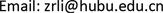﻿ 通信的数学理论与传播的信息科学 Mathematical Theory of Communication and Information Science of Communication

Vol. 08  No. 09 ( 2019 ), Article ID: 32392 , 9 pages
10.12677/ASS.2019.89232

Mathematical Theory of Communication and Information Science of Communication

Zongrong Li1,2, Shaohua Chen1,3, Jianwei Zhang1,4, Aijing Tian1,2

1Huaguang Academy of Information Science, Wuhan Hubei

2College of Computer and Information Engineering, Hubei University, Wuhan Hubei

3Institute of Electronic and Network Publishing, Huazhong University of Science and Technology, Wuhan Hubei

4College of Foreign Languages, Huazhong University of Science and Technology, Wuhan HubeiReceived: Sep. 7th, 2019; accepted: Sep. 22nd, 2019; published: Sep. 29th, 2019ABSTRACT

In his paper “Mathematical Theory of Communication”, Shannon quantitatively describes the physical law of the transmission process of “electric signal” on “channel” by using the mathematical methods of probability statistics and calculus, which fully conforms to the paradigm of natural science and is praised as a scientific model for “quantitative” research of information. However, we believe that the object of Shannon’s “quantitative” research is not “information”, but “message”. Messages are the “carriers” of information, which can be quantified whether they are “signals” or “symbols”. However, the information which is “content” or “meaning” of material carriers has no weight, and does not occupy space; it does not exist in Newton’s time, therefore, we cannot make a physical space-time measurement for it. In this sense, Shannon’s “theory of information” is actual “theory of message”. Before Shannon’s thesis, human beings took “language” as the carrier and successfully constructed the communication history of human society for thousands of years. However, from Wilbur Schramm, people began to study the concepts, principles and methods of communication in accordance with Shannon’s paradigm of “mathematical-physical method”. Although progress has been made in the past hundred years, it is difficult to make a fundamental breakthrough. The authors of this paper point out that the communication science does not belong to natural science, but an information science. We should use the space-time view and scientific paradigm of information science to re-observe and express communication science.

Keywords:Science, Natural Science, Information Science, Space-Time View, Scientific Paradigm, Communication Science

1武汉市华光信息科学研究院，湖北 武汉

2湖北大学计算机与信息工程学院，湖北 武汉

3华中科技大学电子与网络出版研究所，湖北 武汉

4华中科技大学外国语学院，湖北 武汉1. 引言

“二战”以来，施拉姆领导的美国传播学主流坚持经验主义、实证主义和量化研究的思路，服务于现存的政治体制和商业体制。但是，战后的德国法兰克福学派、英国文化研究学派和法国结构主义学派，一波又一波地引进美国；这些学者高扬意识形态批判的旗帜，对于现存的美国体制造成强大的冲击。而真正解放传播学的是北美传播学的媒介环境学派；它有力地矫正了经验学派独霸、批判学派式微的局面，为传播学开辟出一方新的天地。本文作者在重新研究香农理论和现时的传播学之后认为，香农讨论的“通信”是“电信号”在“信道”上的传输过程，属于物理学的领域；他运用概率统计和微积分学的数学方法，定量地描述的对象，不是“信息”，而是“消息”。香农并没有给出“信息”的确切定义，他认为“信息就是一种消息”。消息，作为信息的“载体”，无论是“信号”，还是“符号”都发生在物理学的“时空”中，都是可以量化的。然而，信息是以消息为载体的“内容”或者“含义”，它发生在心理学与文化学“时空”中，因而没有重量、不占据空间，可以“时间无涉”、甚至“时空倒置”，不可进行时空度量。显然，施拉姆认为香农通信的数学理论，既可用于研究电子通讯，也可以用于人类传播，他的出发点和基本思路，从一开始就是错误的。

2. 在香农“通信数学理论”之前，人类“传播实践”已经发展起来了

3. 香农通信的数学理论及其对于传播学建立与发展的“规范性”误导

1948年，香农发表《通信的数学理论》，对于电学通信(有线和无线)的物理模型数量化、公式化，建立香农“信息论”。如图1所示，信源发出消息通过有干扰的信道传递给信宿，构成最简单的通信系统模型。Figure 1. Logical model of a simple communication system

$\left\{\begin{array}{c}X\\ P\left(X\right)\end{array}\right\}=\left\{\begin{array}{c}{a}_{1},{a}_{2},\cdots ,{a}_{i},\cdots ,{a}_{n}\\ P\left({a}_{1}\right),P\left({a}_{2}\right),\cdots ,P\left({a}_{i}\right),\cdots ,P\left({a}_{n}\right)\end{array}\right\},$

$0\le p\left({a}_{i}\right)\le 1,\text{\hspace{0.17em}}\underset{i=1}{\overset{n}{\sum }}P\left({a}_{i}\right);$

$\left\{\begin{array}{c}Y\\ P\left(Y\right)\end{array}\right\}=\left\{\begin{array}{c}{b}_{1},{b}_{2},\cdots ,{b}_{i},\cdots ,{b}_{n}\\ P\left({b}_{1}\right),P\left({b}_{2}\right),\cdots ,P\left({b}_{i}\right),\cdots ,P\left({b}_{n}\right)\end{array}\right\},$

$0\le p\left({b}_{j}\right)\le \text{1},\text{\hspace{0.17em}}\text{\hspace{0.17em}}\underset{j=1}{\overset{m}{\sum }}P\left({b}_{j}\right)=1$

$I\left({a}_{i};{b}_{j}\right)={\mathrm{log}}_{2}\frac{{a}_{i}/{b}_{j}}{P\left({a}_{i}\right)}\text{\hspace{0.17em}}\text{\hspace{0.17em}}\text{\hspace{0.17em}}\left(i=1,2,\cdots ,n;\text{\hspace{0.17em}}j=1,2,\cdots ,m\right)$ 

4. 从“信息论”、“广义信息论”升华为“信息科学”的历程

“信息论”、“广义信息论”和“也叫信息科学”，没有根本性的科学水平的区别，仅仅是学者为它们“科学光环”亮度的“赋值”不同。它们既然遵守同一的物理科学(自然科学)范式，把物质(电流、电压，光、激光)作为研究对象，采用数学物理方法，数量化研究对象，用数学公式表示物质运动、变化的规律性，所以其间的差异就像孪生兄妹一样难被外人察觉。

5. 按照信息科学的“时空观”重新观察“传播”现象

6. 按照信息科学的“科学范式”重新表述“传播学”

7. 结束语

Mathematical Theory of Communication and Information Science of Communication[J]. 社会科学前沿, 2019, 08(09): 1698-1706. https://doi.org/10.12677/ASS.2019.89232

1. 1. W∙施拉姆, W∙波特. 传播学概论[M]. 第二版. 何道宽, 译. 北京: 中国人民大学出版社, 2018: 226.

2. 2. 陈少华. 传播信息学[M]//李宗荣. 理论信息学概论. 北京: 中国科学技术出版社, 2008.

3. 3. 郭晨光. 传播学教程[M]. 第二版. 北京: 中国人民大学出版社, 2019: 9-10.

4. 4. 吴国盛. 科学的历程[M]. 第二版. 北京: 北京大学出版社, 2002.

5. 5. 傅祖芸. 信息论—基础理论与应用[M]. 第二版. 北京: 电子工业出版社, 2018.

6. 6. 鲁晨光. 广义信息论[M]. 合肥: 中国科学技术大学出版社, 1993.

7. 7. 钟义信. 信息科学原理[M]. 第三版. 北京: 北京邮电大学出版社, 2002.

8. 8. 陈运. 信息论与编码[M]. 第二版. 北京: 电子工业出版社, 2012.

9. 9. 田爱景. 传奇学者李宗荣: 原始创新成就超越之梦[J]. 智库时代, 2019(172): 3-4.